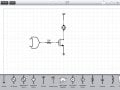# Output of a gate with load attached

Joined Jan 8, 2016
1
Suppose I design an OR gate such that the output voltage is high when either of the input voltage is high. So far so good. But if I add a load at the output, the output voltage can change and can drop to a level of logic zero. How do we get around this problem?

#### bertus

Joined Apr 5, 2008
22,215
Hello,

What kind of gate do you use?
Is it CMOS or TTL?
Cmos can deliver 2 mA at most.
TTL can deliver 20 mA at most.
Read the datasheet of the gat for the exact values.

Bertus

#### GopherT

Joined Nov 23, 2012
8,009

You need to add a "buffer" to the OR gate's output. That will allow the OR gate to function correctly (and not exceed it's designed current limit and, make sure that the load is powered correctly.

You can add a "logic level MOSFET", a transistor that is switched by voltage applied to the gate ( no continuous current flow to activate the switch) and the source-to-drain current can be 10s to 100 amps, depending on the MOSFET you select.#### crutschow

Joined Mar 14, 2008
32,841
What is the value of this "load"?

#### AnalogKid

Joined Aug 1, 2013
10,549
Suppose I design an OR gate such that the output voltage is high when either of the input voltage is high. So far so good. But if I add a load at the output, the output voltage can change and can drop to a level of logic zero. How do we get around this problem?
For any circuit, not just logic devices, if the current required by the load is greater than what can be sunk/sourced by the device, you heed a bigger output stage. For logic circuits this frequently is an external switching transistor that can pull the load up or down depending on the parts.

ak

#### hp1729

Joined Nov 23, 2015
2,304
Suppose I design an OR gate such that the output voltage is high when either of the input voltage is high. So far so good. But if I add a load at the output, the output voltage can change and can drop to a level of logic zero. How do we get around this problem?
Higher capability driver.